International
Tables for
Crystallography
Volume F
Crystallography of biological macromolecules
Edited by M. G. Rossmann and E. Arnold

International Tables for Crystallography (2006). Vol. F, ch. 12.2, pp. 257-258   | 1 | 2 |

## Section 12.2.2. The Patterson function

M. T. Stubbsa* and R. Huberb

aInstitut für Pharmazeutische Chemie der Philipps-Universität Marburg, Marbacher Weg 6, D-35032 Marburg, Germany, and bMax-Planck-Institut für Biochemie, 82152 Martinsried, Germany
Correspondence e-mail:  stubbs@mailer.uni-marburg.de

### 12.2.2. The Patterson function

| top | pdf |

Although the set of measured intensities contains no information regarding the phases, the Fourier transform of the intensities, the so-called Patterson function, contains valuable information. Patterson (1934) showed that the inverse Fourier transform of the intensity,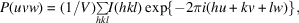is related to the electron density by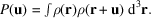The Patterson functionis an autocorrelation function of the density. For every vector u that corresponds to an interatomic vector,will contain a peak (Fig. 12.2.1.1). These are some properties of the Patterson function:

 (1) Every atom makes an interatomic vector' with itself, and therefore the origin peak,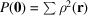, dominates the Patterson function. This origin peak can be removed' through subtraction of the average intensity from I(hkl) before Fourier transformation. (2) For every vector between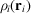and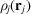, the same value (i.e. their product) is found forto, and so the Patterson map is centrosymmetric. (3) For a structure consisting of n atoms, there are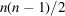cross vectors, and so the Patterson function is extremely crowded.

For simple crystals, the Patterson map can be used to solve the structure directly. For macromolecular structures, the Patterson map provides a vehicle for solving the phase problem.

If the crystal contains rotational symmetry elements, then the cross vectors betweenand its symmetry mate lie on a plane perpendicular to the symmetry axis – the Harker section (Harker, 1956). By way of example, the space grouphas two symmetry-related positions (Fig. 12.2.2.1),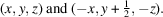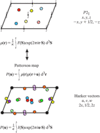Figure 12.2.2.1 | top | pdf |The Patterson map with symmetry. When the crystal unit cell contains more than one molecule, then additional cross vectors will be formed between differing molecules. If these are related by crystallographic symmetry, there is a geometrical relationship between cross peaks. In this diagram, the peaks of Fig. 12.2.1.1are supplemented by those between atoms of symmetry-related molecules. The red, yellow and blue peaks of the resulting Patterson function represent those between same atoms (i.e. red to red, yellow to yellow and blue to blue) related by symmetry. These peaks are found on a Harker section.

Cross vectors between symmetry-related points will therefore have the form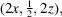i.e. all cross vectors lie on the plane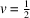. For space group, the general coordinates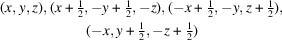give rise to cross vectors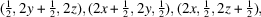i.e. there are three Harker sections:,and. Peaks occurring on the Harker sections must reduce to a self-consistent set of coordinates (x, y, z), allowing reconstruction of the atomic positions.

If we have two isomorphous (see below) data sets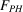and, then the difference in the two Patterson functions,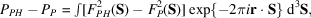will deliver information about the heavy-atom structure. Such a difference function gives rise to non-negligible peaks arising from interference between theandterms, however (Perutz, 1956). Rossmann (1960) showed that these interference terms could be reduced through calculation of the modified Patterson function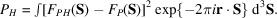In the case of a single-site derivative, peaks should occur only at the Harker vectors corresponding to the heavy-atom position. Even so, there is a choice of positions for the heavy atom: e.g., in thecase, coordinates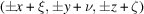, where ξ, ν and ζ can each take the value 0 or, will all give rise to the same Harker vectors. This in itself is not a problem, relating to equivalent choices of origin and of handedness, but has important ramifications for multisite derivatives or multiple isomorphous replacement (see below).

If there is more than one site, then there will be two sets of peaks: one set corresponding to the Harker sections (self-vector set) and one set corresponding to the difference vectors between different heavy-atom sites (the cross-vector set). In this case, the choice of one heavy-atom position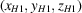determines the origin and the handedness to which all other peaks must correspond. Thus, in theexample, only one cross vector will occur for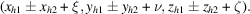An alternative to the Harker-vector approach is Patterson-vector superposition (Sheldrick et al., 1993; Richardson & Jacobson, 1987). The Patterson map contains several images of the structure that have been shifted by interatomic vectors (Fig. 12.2.2.2). If this structure is relatively simple (as is to be hoped for in a `normal' heavy-atom derivative), then it should be possible to deconvolute the superimposed structures by vector shifts (Buerger, 1959).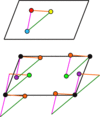Figure 12.2.2.2 | top | pdf |The vector superposition method. The Patterson map of Fig. 12.2.1.1can be regarded as the superposition of the structure (and its inverse), with each of its atoms placed alternately at the origin. By shifting each peak of the Patterson function to the origin and calculating the correlation of all remaining peaks with the unshifted map, it is possible to deconvolute the Patterson function.

### References

Buerger, M. J. (1959). Vector space. New York: Wiley.Google Scholar
Harker, D. (1956). The determination of the phases of the structure factors of non-centrosymmetric crystals by the method of double isomorphous replacement. Acta Cryst. 9, 1–9.Google Scholar
Patterson, A. L. (1934). A Fourier series method for the determination of the components of interatomic distances in crystals. Phys. Rev. 46, 372–376.Google Scholar
Perutz, M. F. (1956). Isomorphous replacement and phase determination in non-centrosymmetric space groups. Acta Cryst. 9, 867–873.Google Scholar
Richardson, J. W. & Jacobson, R. A. (1987). In Patterson and Pattersons, edited by J. P. Glusker, B. K. Patterson & M. Rossi. Oxford University Press.Google Scholar
Rossmann, M. G. (1960). The accurate determination of the position and shape of heavy-atom replacement groups in proteins. Acta Cryst. 13, 221–226.Google Scholar
Sheldrick, G. M., Dauter, Z., Wilson, K. S., Hope, H. & Sieker, L. C. (1993). The application of direct methods and Patterson interpretation to high-resolution native protein data. Acta Cryst. D49, 18–23.Google Scholar